The temperature (T) dependence of resistivity ($\rho$) of a semiconductor is represented by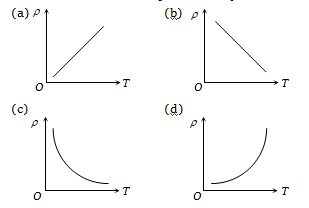Concept Questions :-

Types of semiconductors
High Yielding Test Series + Question Bank - NEET 2020

Difficulty Level:

In a forward biased PN-junction diode, the potential barrier in the depletion region is of the form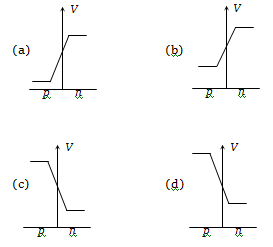Concept Questions :-

PN junction
High Yielding Test Series + Question Bank - NEET 2020

Difficulty Level:

Different voltages are applied across a P-N junction and the currents are measured for each value. Which of the following graphs is obtained between voltage and current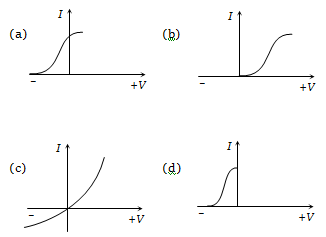Concept Questions :-

PN junction
High Yielding Test Series + Question Bank - NEET 2020

Difficulty Level:

If the following input signal is sent through a PN-junction diode, then the output signal across ${\mathrm{R}}_{\mathrm{L}}$ will be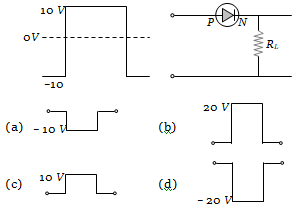Concept Questions :-

PN junction
High Yielding Test Series + Question Bank - NEET 2020

Difficulty Level:

The curve between charge density and distance near P-N junction will be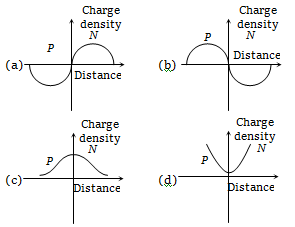Concept Questions :-

PN junction
High Yielding Test Series + Question Bank - NEET 2020

Difficulty Level:

The resistance of a germanium junction diode whose is shown in figure is $\left({\mathrm{V}}_{\mathrm{k}}=0.3\mathrm{V}\right)$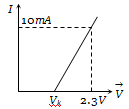(a) 5 k$\mathrm{\Omega }$
(b) 0.2 k$\mathrm{\Omega }$
(c) 2.3 k$\mathrm{\Omega }$
(d) $\left(\frac{10}{2.3}\right)\mathrm{k\Omega }$

Concept Questions :-

PN junction
High Yielding Test Series + Question Bank - NEET 2020

Difficulty Level:

In the half-wave rectifier circuit shown. Which one of the following wave forms is true for ${\mathrm{V}}_{\mathrm{CD}}$, the output across C and D?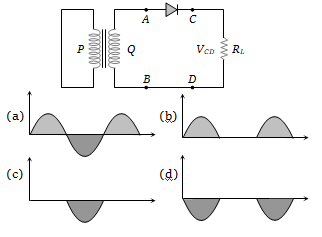Concept Questions :-

Rectifier
High Yielding Test Series + Question Bank - NEET 2020

Difficulty Level:

A full-wave rectifier circuit along with the input and output voltages is shown in the figure.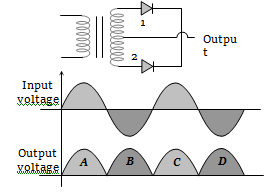The contribution to the output voltage from diode – 2 is :

(a) A, C                       (b) B, D
(c) B, C                       (d) A, D

Concept Questions :-

Rectifier
High Yielding Test Series + Question Bank - NEET 2020

Difficulty Level:

The i-V characteristic of a P-N junction diode is shown below. The approximate dynamic resistance of the P-N junction when a forward bias of 2 volt is applied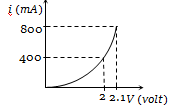(a) 1 $\mathrm{\Omega }$
(b) 0.25 $\mathrm{\Omega }$
(c) 0.5 $\mathrm{\Omega }$
(d) 5 $\mathrm{\Omega }$

Concept Questions :-

PN junction
High Yielding Test Series + Question Bank - NEET 2020

Difficulty Level:

The given figure shows the wave forms for two inputs A and B and that for the output Y of a logic circuit. The logic circuit is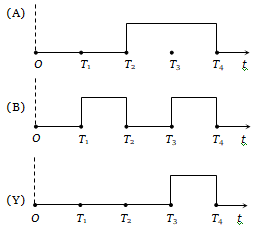(a) An AND gate                   (b) An OR gate
(c) A NAND gate                   (d) An NOT gate

Concept Questions :-

Logic gates